# Descriptive Statistics with Basic Data Visualization in R programming

R programming language is an extremely versatile and user friendly software for programming. It is commonly used for statistical analysis. Lets go over some of the most basic yet frequently used functions in R.

Functions covered:

• Basic Statistical Functions
• Other important functions in different libraries
• Important Plots in R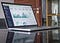Photo by Carlos Muza on Unsplash

# Basic Statistical Functions

You must’ve heard a lot of statistical concepts used in Descriptive Statistics. Descriptive statistics helps us understand the key features that describe a given dataset. This vast topic includes concepts like the Mean of the sample, Median, Mode, Skewness, Kurtosis and Moments (raw and central). …

# Data Science Misconceptions

Given below are a series of statements that I have heard over time! They might make sense initially, but lets analyze it deeper to come to a logical conclusion. If the statements are in fact untrue, lets see why!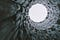Photo by Artem Maltsev on Unsplash
• “Once I learn Python /R programming / Java / MATLAB and other sophisticated programming, I can easily get a role in Data science.”

This is like, expecting to become a chef by just knowing the recipes at heart. …

# Statistical Distributions for a career in Data Science

Like we discussed, you need to know basics of statistics to be able to analyze the data better. And one topic of statistics, the most important in Data science industry, is the Concept of Distributions.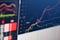Photo by Nicholas Cappello on Unsplash

What are you waiting for! Lets dive into the important theory needed in data science and its R application.

But first lets understand, what does it mean by Distributions in Statistics? Well, there are certain parameters of the distribution, this makes each distribution unique from the other. It is a pre-determined set of observations that are likely to occur based on the pattern that population…

# Must’ve skills for a career in Data Science and their free-resources

In my previous article, we went over some of the foundational topics used by Data Science professionals. In order to develop the must-have’s for this profession, we must use our time to the fullest and upskill.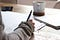Photo by Green Chameleon on Unsplash

# Must’ve skills

Whenever someone applies for a job opportunity, there are always a set of skills that the hiring team looks for, could be some specific softer skills or some technical skills. And so, we ought to prepare well with those skills. We would be able to upskill better, once we know the various stops of a Data Scientist.

# Test for Heteroscedasticity, Multicollinearity and Autocorrelation

In the articles earlier, we understood the importance of observing the three behaviors in the model: Homoscedasticity, Multicollinearity and Autocorrelation.

We would be going over the concept and R application of most used tests in multiple regression modeling. The list is as follows: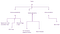Image by author
1. Breush-Pagan test
2. VIF (Variance Inflation Factor)
3. Runs test
4. Box-Cox transformation to address heteroscedasticity.

# Breush-Pagan test

Lets understand this by using the model built in the earlier article and applying the test for heteroscedasticity in the model. Here we set the following hypothesis for the test.
H0: There is constant variation in the model, i.e., …

# Multiple Linear Regression

In the last two articles, we explored the concept of Simple Linear Regression Model (i.e., regression involving two variables). Although, the practical situations demand much more complexity, for almost all the situations when we apply this concept in real life, we have numerous variables (which might or might not affect the outcome variable significantly). Let’s learn how to build and optimize our Multiple Linear regression model (model with more than one explanatory variables).

Example: Lets consider the following dataset using which, we intend to explain the price of the flat (Y) using the following explanatory variables: Number of Bedrooms, Number…

# Linear Regression Model-Part 2

In my previous article, we went over the model fitting for the given data of percentage of hardwood pulp in the paper(X) and the tensile strength of the paper (Y).

Once we have established the significance of the regression model and the estimated of the population parameters of the model, we then have to analyze the trends of the residuals to verify the assumptions of the linear regression model. We run the following R code to obtain a series if insightful plots as discussed below.

Note: The assumptions of the linear regression plots cannot be completely met, but we are…

# Simple Linear Regression Modeling-Part 1

Regression Analysis is one of the most acknowledged and useful tools of statistics. It is one of the most efficient ways to understand the relation between certain variables, while being able to make logical predictions for the future.

Lets understand Simple linear regression with an example and R codes. But first, we should know why is it being referred as “simple” linear regression. Well, this is because we would be studying the relation between only two variables, one dependent and one independent (clearly explained with the example below).

Example
Consider the example given below:
Suppose we wish to study the impact of…

# Testing for Normality

In my previous article, we went over the single variable hypothesis testing. Likewise, we can apply the tests for the double variable testing like, testing for the significance of the difference of means, testing for the difference of population variances and others.

But now, we would move over the topic of testing for the normality of any form of sample (data). But first lets understand what does Normality mean? When we use normality in context of hypothesis testing, we mean to test if the sample seems to originate from a normal distribution, i.e., …

# Test Statistics for Hypothesis Testing in Statistics with Functions in R Programming

In my previous article, we talked about the terminology in the statistical world of hypothesis testing.

Moving on, once we have our hypothesis about the population parameters (based on the sample) in place, we need some sophisticated ways to practically support one claim (either H0 or H1) by using statistical inference, Based on the type of statistics, i.e., population mean, population variance, population correlations, testing for normality or testing for attributes, we have separate test statistics for each hypothesis testing.

Lets go over some of the commonly used test statistics in hypothesis testing and their R-programming codes:

1. Testing for the…